Numerical Analysis & Statistics: MATLAB, R, NumPy, Julia

a side-by-side reference sheet

sheet two: tables | import and export | relational algebra | aggregation

tables
matlabrnumpyjulia
construct from column arrayssx = {'F' 'F' 'F' 'M' 'M' 'M'}
ht = [69 64 67 68 72 71]
wt = [148 132 142 149 167 165]
cols = {'sx', 'ht', 'wt'}
people = table(sx', ht', wt', 'VariableNames', cols)
# gender, height, weight of some people
# in inches and lbs:

sx = c("F", "F", "F", "M", "M", "M")
ht = c(69, 64, 67, 68, 72, 71)
wt = c(148, 132, 142, 149, 167, 165)
people = data.frame(sx, ht, wt)
sx = ['F', 'F', 'F', 'F', 'M', 'M']
ht = [69, 64, 67, 66, 72, 70]
wt = [150, 132, 142, 139, 167, 165]
people = pd.DataFrame({'sx': sx, 'ht': ht, 'wt': wt})
construct from row dictionariesrows = [
{'sx': 'F', 'ht': 69, 'wt': 150},
{'sx': 'F', 'ht': 64, 'wt': 132},
{'sx': 'F', 'ht': 67, 'wt': 142},
{'sx': 'F', 'ht': 66, 'wt': 139},
{'sx': 'M', 'ht': 72, 'wt': 167},
{'sx': 'M', 'ht': 70, 'wt': 165}]
people = pd.DataFrame(rows)
sizeheight(people)
width(people)
nrow(people)
ncol(people)

# number of rows and cols in 2-element vector:
dim(people)
len(people)
len(people.columns)
column names as arraypeople.Properties.VariableNamesnames(people)
colnames(people)
returns Index object:
people.columns
access column as arraypeople.ht
people.(2)
# vectors:
people$ht people[,2] people[['ht']] people[] # 1 column data frame: people people['ht'] # if name does not conflict with any DataFrame attributes: people.ht access row as tuplepeople(1,:)# 1 row data frame: people[1, ] # list: as.list(people[1, ]) people.ix access datum% height of 1st person: people(1,2) # height of 1st person: people[1,2] people.get_value(0, 'ht') order rows by columnsortrows(people, 'ht')people[order(people$ht), ]people.sort(['ht'])
order rows by multiple columnssortrows(people, {'sx', 'ht'})people[order(people$sx, people$ht), ]people.sort(['sx', 'ht'])
order rows in descending ordersortrows(people, 'ht', 'descend')people[order(-people$ht), ]people.sort('ht', ascending=[False]) limit rows people(1:3, :)people[seq(1, 3), ]people[0:3] offset rows people(4:6, :)people[seq(4, 6), ]people[3:] reshapepeople$couple = c(1, 2, 3, 1, 2, 3)
reshape(people, idvar="couple", direction="wide",
timevar="sx", v.names=c("ht", "wt"))
remove rows with null fieldssx = c('F', 'F', 'M', 'M')
wt = c(120, NA, 150, 170)

df = data.frame(sx, wt)
df2 = na.omit(df)
attach columns# put columns ht, wt, and sx
# in variable name search path:

attach(people)
sum(ht)

# alternative which doesn't put columns in
# search path:

with(people, sum(ht))
none
detach columns

detach(people)none
spreadsheet editorcan edit data, in which case return value of edit must be saved
people = edit(people)
none
import and export
matlabrnumpyjulia
import tab delimited# first row defines variable names:

# file suffix must be .txt, .dat, or .csv
# first row defines variable names:
# first row defines column names:
import csv

% first row defines variable names:
# first row defines variable names:
# first row defines column names:
'Delimiter', ':',
sep=':',
comment.char='#')
# $grep -v '^#' /etc/passwd > /tmp/passwd df = pd.read_table('/tmp/passwd', sep=':', header=None) set column separator to whitespacedf = read.delim('/path/to.txt', sep='')df = read_table('/path/to.txt', sep='\s+') set quote character# default quote character for both read.csv and read.delim # is double quotes. The quote character is escaped by doubling it. # use single quote as quote character: df = read.csv('/path/to/single-quote.csv', quote="'") # no quote character: df = read.csv('/path/to/no-quote.csv', quote="") Both read_table and read_csv use double quotes as the quote character and there is no way to change it. A double quote can be escaped by doubling it. import file w/o header# column names are V1, V2, … read.delim('/etc/passwd', sep=':', header=FALSE, comment.char='#') #$ grep -v '^#' /etc/passwd > /tmp/passwd
#
# column names are X0, X1, …

df.Properties.VariableNames = {'ht', 'wt', 'age'}
col.names=c('ht', 'wt', 'age'))
names=['ht', 'wt', 'age'])
set column types# possible values: NA, 'logical', 'integer', 'numeric',
# 'complex', 'character', 'raw', 'factor', 'Date',
# 'POSIXct'
#
# If type is set to NA, actual type will be inferred to be
# 'logical', 'integer', 'numeric', 'complex', or 'factor'
#

colClasses=c('integer', 'numeric', 'character'))
colClasses=c('integer', 'logical', 'character'),
na.strings=c('nil'))
na_values=['nil'])
change decimal markdf = read.csv('/path/to.csv', dec=',')
recognize thousands separatornonedf = read_csv('/path/to.csv', thousands='.')
unequal row length behaviorMissing fields will be set to NA unless fill is set to FALSE. If the column is of type character then the fill value is an empty string ''.

If there are extra fields they will be parsed as an extra row unless
flush is set to FALSE
sep=':',
comment.char='#')
none

# rows to skip can be specified individually:

# hierarchical index:
export tab delimitedwrite.table(df, '/tmp/data.tab', sep='\t')
export csv

# first column contains row names unless row.names
# set to FALSE

write.csv(df, '/path/to.csv', row.names=F)
relational algebra
matlabrnumpyjulia
project columns by namepeople(:, {'sx', 'ht'})people[c('sx', 'ht')]people[['sx', 'ht']]
project columns by positionpeople(:, [1 2])people[c(1, 2)]
project expression# convert to cm and kg:
transform(people, ht=2.54*ht, wt=wt/2.2)
project all columnspeople(people.ht > 66, :)people[people$ht > 66, ] rename columnscolnames(people) = c('gender', 'height', 'weight') access sub data frame# data frame of first 3 rows with # ht and wt columns reversed: people[1:3, c(1, 3, 2)] select rowspeople(people.ht > 66, :)subset(people, ht > 66) people[people$ht > 66, ]
people[people['ht'] > 66]
select distinct rowsunique(people(:,{'sx'}))unique(people[c('sx')])
split rows# class(x) is list:
x = split(people, people$sx == 'F') # data.frame only containing females: x$T
sep=':',
comment.char='#',
col.names=c('name', 'passwd', 'uid', 'gid', 'gecos',
'home', 'shell'))

sep=':',
comment.char='#',
col.names=c('name', 'passwd', 'gid', 'members'))

merge(pw, grp, by.x='gid', by.y='gid')
# $grep -v '^#' /etc/passwd > /tmp/passwd #$ grep -v '^#' /etc/group > /tmp/group

pw = pd.read_table('/tmp/passwd', sep=':', header=None, names=['name', 'passwd', 'uid', 'gid', 'gecos', 'home', 'shell'])

pd.merge(pw, grp, left_on='gid', right_on='gid')
nulls as join values
left joinmerge(pw, grp, by.x='gid', by.y='gid', all.x=T)pd.merge(pw, grp, left_on='gid', right_on='gid', how='left')
full joinmerge(pw, grp, by.x='gid', by.y='gid', all=T)pd.merge(pw, grp, left_on='gid', right_on='gid', how='outer')
antijoinpw[!(pw$gid %in% grp$gid), ]
cross joinmerge(pw, grp, by=c())
aggregation
matlabrnumpyjulia
group by columngrouped = people.groupby('sx')
grouped.aggregate(np.max)['ht']
multiple aggregated valuesgrouped = people.groupby('sx')
grouped.aggregate(np.max)[['ht', 'wt']]
group by multiple columns
aggregation functions
nulls and aggregation functions
vectors
matlabrnumpyjulia
vector literal

same as arraysame as arraysame as arraysame as array
element-wise arithmetic operators+ - .* ./+ - * /+ - * /+ - .* ./
result of vector length mismatchraises errorvalues in shorter vector are recycled; warning if one vector is not a multiple length of the otherraises ValueErrorDimensionMismatch
scalar multiplication3 * [1, 2, 3]
[1, 2, 3] * 3
3 * c(1, 2, 3)
c(1, 2, 3) * 3
3 * np.array([1, 2, 3])
np.array([1, 2, 3]) * 3
3 * [1, 2, 3]
[1, 2, 3] * 3
dot productdot([1, 1, 1], [2, 2, 2])c(1, 1, 1) %*% c(2, 2, 2)v1 = np.array([1, 1, 1])
v2 = np.array([2, 2, 2])
np.dot(v1, v2)
dot([1, 1, 1], [2, 2, 2])
cross productcross([1, 0, 0], [0, 1, 0])v1 = np.array([1, 0, 0])
v2 = np.array([0, 1, 0])
np.cross(v1, v2)
cross([1, 0, 0], [0, 1, 0])
normsnorm([1, 2, 3], 1)
norm([1, 2, 3], 2)
norm([1, 2, 3], Inf)
vnorm = function(x, t) {
norm(matrix(x, ncol=1), t)
}

vnorm(c(1, 2, 3), "1")
vnorm(c(1, 2, 3), "E")
vnorm(c(1, 2, 3), "I")
v = np.array([1, 2, 3])
np.linalg.norm(v, 1)
np.linalg.norm(v, 2)
np.linalg.norm(v, np.inf)
v = [1, 2, 3]

norm(v, 1)
norm(v, 2)
norm(v, Inf)
matrices
matlabrnumpyjulia
literal or constructor% row-major order:
A = [1, 2; 3, 4]
B = [4 3
2 1]
# column-major order:
A = matrix(c(1, 3, 2, 4), 2, 2)
B = matrix(c(4, 2, 3, 1), nrow=2)

# row-major order:
A = matrix(c(1, 2, 3, 4), nrow=2, byrow=T)
# row-major order:
A = np.matrix([[1, 2], [3, 4]])
B = np.matrix([[4, 3], [2, 1]])
A = [1 2; 3 4]
B = [4 3; 2 1]
constant matrices
all zeros, all ones
zeros(3, 3) or zeros(3)
ones(3, 3) or ones(3)
matrix(0, 3, 3)
matrix(1, 3, 3)
np.matrix(np.ones([3, 3]))
np.matrix(np.zeros([3, 3]))
zeros(Float64, (3, 3))
ones(Float64, (3, 3))
diagonal matrices
and identity
diag([1, 2, 3])
% 3x3 identity:
eye(3)
diag(c(1, 2, 3)
# 3x3 identity:
diag(3)
np.diag([1, 2, 3])
np.identity(3)
diagm([1, 2, 3])
eye(3)
matrix by formulai = ones(10, 1) * (1:10)
j = (1:10)' * ones(1, 10)
% use component-wise ops only:
1 ./ (i + j - 1)
dimensionsrows(A)
columns(A)
dim(A)
dim(A)
nrows, ncols = A.shapenrows, ncols = size([1 2 3; 4 5 6])
element access

A(1, 1)A[1, 1]A[0, 0]A[1, 1]
row access

A(1, 1:2)A[1, ]AA[1, :]
column access

A(1:2, 1)A[, 1]A[:, 0]A[:, 1]
submatrix accessC = [1, 2, 3; 4, 5, 6; 7, 8, 9]
C(1:2, 1:2)
C = matrix(seq(1, 9), 3, 3, byrow=T)
C[1:2, 1:2]
A = np.matrix(range(1, 10)).reshape(3, 3)
A[:2, :2]
reshape(1:9, 3, 3)[1:2, 1:2]
scalar multiplication3 * A
A * 3
also:
3 .* A
A .* 3
3 * A
A * 3
3 * A
A * 3
3 * [1 2; 3 4]
[1 2; 3 4] * 3
element-wise operators.+ .- .* ./+ - * /+ - np.multiply() np.divide()+ - .* ./
multiplication

A * BA %*% Bnp.dot(A, B)A * B
powerA ^ 3

% power of each entry:
A .^ 3
A ** 3A ^ 3

# power of each entry:
A .^ 3
kronecker product

kron(A, B)kronecker(A, B)np.kron(A, B)kron(A, B)
comparisonall(all(A == B))
any(any(A ~= B))
all(A == B)
any(A != B)
np.all(A == B)
np.any(A != B)
A == B
A != B
normsnorm(A, 1)
norm(A, 2)
norm(A, Inf)
norm(A, 'fro')
norm(A, "1")
??
norm(A, "I")
norm(A, "F")
norm(A, 1)
norm(A, 2)
norm(A, Inf)
# Froebenius norm:
vecnorm(A, 2)
transpose

transpose(A)t(A)A.transpose()transpose([1 2; 3 4])
conjugate transposeA = [1i, 2i; 3i, 4i]
A'
A = matrix(c(1i, 2i, 3i, 4i), nrow=2, byrow=T)
Conj(t(A))
A = np.matrix([[1j, 2j], [3j, 4j]])
A.conj().transpose()
[1im 2im; 3im 4im]'
ctranspose([1im 2im; 3im 4im])
inverse

inv(A)solve(A)np.linalg.inv(A)inv([1 2; 3 4])
pseudoinverseA = [0 1; 0 0]

pinv(A)
install.packages('corpcor')
library(corpcor)

A = matrix(c(0, 0, 1, 0), nrow=2)
pseudoinverse(A)
A = np.matrix([[0, 1], [0, 0]])

np.linalg.pinv(A)
pinv([0 1; 0 0])
determinant

det(A)det(A)np.linalg.det(A)det(1 2; 3 4])
trace

trace(A)sum(diag(A))A.trace()trace([1 2; 3 4])
eigenvalues

eig(A)eigen(A)$valuesnp.linalg.eigvals(A)eigvals(A) eigenvectors[evec, eval] = eig(A) % each column of evec is an eigenvector % eval is a diagonal matrix of eigenvalues eigen(A)$vectorsnp.linalg.eig(A)eigvecs(A)
singular value decompositionX = randn(10)

[u, d, v] = svd(X)
X = matrix(rnorm(100), nrow=10)
result = svd(X)

# singular values:
result$d # matrix of eigenvectors: result$u

# unitary matrix:
result$v np.linalg.svd(np.random.randn(100).reshape(10, 10))X = randn(10, 10) u, s, v = svds(X) solve system of equationsA \ [2;3]solve(A, c(2, 3))np.linalg.solve(A, [2, 3])[1 2; 3 4] \ [2; 3] sparse matrices matlabrnumpyjulia sparse matrix construction% 100x100 matrix with 5 at (1, 1) and 4 at (2, 2): X = sparse([1 2], [1 2], [5 4], 100, 100) X = spMatrix(100, 100, c(1, 2), c(1, 2), c(5, 4))import scipy.sparse as sparse row, col, val = [5, 4], [1, 2], [1, 2] X = sparse.coo_matrix((val, (row, col)), shape=(100, 100)) sparse matrix decomposition[rows, cols, vals] = find(X) % just the values: nonzeros(X) sparse identity matrix% 100x100 identity: speye(100) sparse.identity(100) # not square; ones on diagonal: sparse.eye(100, 200) dense matrix to sparse matrix and back X = sparse([1 0 0; 0 0 0; 0 0 0]) X2 = full(X) imoprt scipy.sparse as sparse A = np.array([[1, 0, 0], [0, 0, 0], [0, 0, 0]]) X = sparse.coo_matrix(A) X2 = X.todense() sparse matrix storage% is storage sparse: issparse(X) % memory allocation in bytes: nzmax(X) % number of nonzero entries: nnz(X) # memory allocation in bytes: object.size(X) import scipy.sparse as sparse sparse.issparse(X) optimization matlabrnumpyjulia linear minimization% download and install cvx: cvx_begin variable x1; variable x2; variable x3; minimize x1 + x2 + x3; subject to x1 + x2 >= 1; x2 + x3 >= 1; x1 + x3 >= 1; cvx_end; % 'Solved' in cvx_status % argmin in x1, x2, x3 % minval in cvx_optval # install.packages('lpSolve') require(lpSolve) obj = c(1, 1, 1) A = matrix(c(1, 1, 0, 0, 1, 1, 1, 0, 1), nrow=3, byrow=T) dir = c(">=", ">=", ">=") rhs = c(1, 1, 1) result = lp("min", obj, A, dir, rhs) # 0 in result$status
# argmin in result$solution # minval in result$objval
# sudo pip install cvxopt
from cvxopt.modeling import *

x1 = variable(1, 'x1')
x2 = variable(1, 'x2')
x3 = variable(1, 'x3')
c1 = (x1 + x2 >= 1)
c2 = (x1 + x3 >= 1)
c3 = (x2 + x3 >= 1)
lp = op(x1 + x2 + x3, [c1, c2, c3])
lp.solve()

# 'optimal' in lp.status
# argmin in x1.value, x2.value,
#   x3.value

# minval in lp.objective.value()
decision variable vectorcvx_begin
variable x(3);
minimize sum(x);
subject to
x(1) + x(2) >= 1;
x(2) + x(3) >= 1;
x(1) + x(3) >= 1;
cvx_end;
# decision variables must be an array# sudo pip install cvxopt
from cvxopt.modeling import *

x = variable(3, 'x')
c1 = (x + x >= 1)
c2 = (x + x >= 1)
c3 = (x + x >= 1)
lp = op(x + x + x, [c1, c2, c3])
lp.solve()
linear maximizationcvx_begin
variable x(3);
maximize sum(x);
subject to
x(1) + x(2) <= 1;
x(2) + x(3) <= 1;
x(1) + x(3) <= 1;
cvx_end;
# install.packages('lpSolve')
require(lpSolve)

obj = c(1, 1, 1)
A = matrix(c(1, 1, 0, 0, 1, 1, 1, 0, 1),
nrow=3, byrow=T)
dir = c("<=", "<=", "<=")
rhs = c(1, 1, 1)
result = lp("max", obj, A, dir, rhs)
# None; negate objective function before
# solving; negate optimal value which
# is found.
constraint in variable declarationcvx_begin
variable x(3) nonnegative;
minimize 10*x(1) + 5*x(2) + 4*x(3);
subject to
x(1) + x(2) + x(3) >= 10;
cvx_end
# none; but note that variables are assumed
# to be nonnegative
# none
unbounded behaviorcvx_begin
variable x(3);
maximize sum(x);
cvx_end

% Inf in cvx_optval
% 'Unbounded' in cvx_status
# install.packages('lpSolve')
require(lpSolve)

obj = c(1, 1, 1)
A = matrix(c(1, 1, 1), nrow=1, byrow=T)
dir = c(">=")
rhs = c(1)
result = lp("max", obj, A, dir, rhs)

# result$status is 3 # sudo pip install cvxopt from cvxopt.modeling import * x = variable(3, 'x') c1 = (x >= 0) c2 = (x >= 0) c3 = (x <= 0) lp = op(x + x + x, [c1, c2, c3]) lp.solve() # lp.status is 'dual infeasible' infeasible behaviorcvx_begin variable x(3) nonnegative; maximize sum(x); subject to x(1) + x(2) + x(3) < -1; cvx_end % -Inf in cvx_optval % 'Infeasible' in cvx_status # install.packages('lpSolve') require(lpSolve) obj = c(1, 1, 1) A = matrix(c(1, 1, 1), nrow=1, byrow=T) dir = c("<=") rhs = c(-1) result = lp("min", obj, A, dir, rhs) # result$status is 2
# sudo pip install cvxopt
from cvxopt.modeling import *

x = variable(3, 'x')
c1 = (x >= 0)
c2 = (x >= 0)
c3 = (x >= 0)
c4 = (x + x + x <= -1)
lp = op(x + x + x, [c1, c2, c3, c4])
lp.solve()

# lp.status is 'primal infeasible'
integer decision variable% requires Optimization Toolbox:
f = [1 1 1]
A = [-1 -1 0; -1 0 -1; 0 -1 -1;
-1 0 0; 0 -1 0; 0 0 -1]
b = [-1 -1 -1 0 0 0]
% 2nd arg indicates integer vars
[x opt flag] = intlinprog(f, [1 1 1], A, b)

% if solution found, flag is 1
% x is argmin
% opt is optimal value
# install.packages('lpSolve')
require(lpSolve)

obj = c(1, 1, 1)
A = matrix(c(1, 1, 0, 0, 1, 1, 1, 0, 1),
nrow=3, byrow=T)
dir = c(">=", ">=", ">=")
rhs = c(1, 1, 1)
result = lp("min", obj, A, dir, rhs,
int.vec=c(1, 1, 1))
binary decision variable# install.packages('lpSolve')
require(lpSolve)

obj = c(1, 1, 1)
A = matrix(c(1, 1, 0, 0, 1, 1, 1, 0, 1),
nrow=3, byrow=T)
dir = c(">=", ">=", ">=")
rhs = c(1, 1, 1)
result = lp("min", obj, A, dir, rhs,
binary.vec=c(1, 1, 1))
# integer solver not provided by default
polynomials
matlabrnumpyjulia
exact polynomial fitx = [1 2 3 4]
y = [3 9 2 1]
% polynomial coefficient array:
p = polyfit(x, y, 3)

% plot polynomial:
xx = -10:.1:10
yy = polyval(p, xx)
plot(xx, yy)
exact polynomial fit with derivative values
piecewise polynomial fitcubic spline
f = spline(1:20, normrnd(0, 1, 1, 20))
x = 1:.1:20
plot(x, ppval(f, x))
f = splinefun(rnorm(20))
x = seq(1, 20, .1)
plot(x, f(x), type="l")
underdetermined polynomail fit
overdetermined polynomial fit
multivariate polynomial fit
descriptive statistics
matlabrnumpyjulia
first moment statisticsx = [1 2 3 8 12 19]

sum(x)
mean(x)
x = c(1,2,3,8,12,19)

sum(x)
mean(x)
x = [1,2,3,8,12,19]

sp.sum(x)
sp.mean(x)
x = [1 2 3 8 12 19]

sum(x)
mean(x)
second moment statisticsstd(x, 1)
var(x, 1)
n = length(x)

sd(x) * sqrt((n-1)/n)
var(x) * (n-1)/n
sp.std(x)
sp.var(x)
second moment statistics for samplesstd(x)
var(x)
sd(x)
var(x)
n = float(len(x))

sp.std(x) * math.sqrt(n/(n-1))
sp.var(x) * n/(n-1)
std(x)
var(x)
skewnessOctave uses sample standard deviation to compute skewness:
skewness(x)
install.packages('moments')
library('moments')

skewness(x)
stats.skew(x)
kurtosisOctave uses sample standard deviation to compute kurtosis:
kurtosis(x)
install.packages('moments')
library('moments')

kurtosis(x) - 3
stats.kurtosis(x)
nth moment and nth central momentn = 5

moment(x, n)
moment(x, n, "c")
install.packages('moments')
library('moments')

n = 5
moment(x, n)
moment(x, n, central=T)
n = 5

??
stats.moment(x, n)
modemode([1 2 2 2 3 3 4])samp = c(1,2,2,2,3,3,4)
names(sort(-table(samp)))
stats.mode([1,2,2,2,3,3,4])
quantile statisticsmin(x)
median(x)
max(x)
iqr(x)
quantile(x, .90)
min(x)
median(x)
max(x)
IQR(x)
quantile(x, prob=.90)
min(x)
sp.median(x)
max(x)
??
stats.scoreatpercentile(x, 90.0)
bivariate statistiscs
correlation, covariance
x = [1 2 3]
y = [2 4 7]

cor(x, y)
cov(x, y)
x = c(1,2,3)
y = c(2,4,7)

cor(x, y)
cov(x, y)
x = [1,2,3]
y = [2,4,7]

stats.linregress(x, y)
??
correlation matrixx1 = randn(100, 1)
x2 = 0.5 * x1 + randn(100, 1)
x3 = 0.1 * x1 + 0.1 * x2 + 0.1 * randn(100, 1)

corr([x1 x2 x3])
x1 = rnorm(100)
x2 = x1 + 0.5 * rnorm(100)
x3 = 0.3 * x1 + 0.1 * 2 + 0.1 * rnorm(100)

cor(cbind(x1, x2, x3))
data set to frequency tablex = c(1,2,1,1,2,5,1,2,7)
tab = table(x)
frequency table to data setrep(as.integer(names(tab)),
unname(tab))
binx = c(1.1, 3.7, 8.9, 1.2, 1.9, 4.1)
xf = cut(x, breaks=c(0, 3, 6, 9))
# bins are (0, 3], (3, 6], and (6, 9]:
bins = tapply(x, xf, length)
distributions
matlabrnumpyjulia
binomial
density, cumulative, quantile,
sample of 10
binopdf(x, n, p)
binocdf(x, n, p)
binoinv(y, n, p)
binornd(n, p, 1, 10)
dbinom(x, n, p)
pbinom(x, n, p)
qbinom(y, n, p)
rbinom(10, n, p)
stats.binom.pmf(x, n, p)
stats.binom.cdf(x, n, p)
stats.binom.ppf(y, n, p)
stats.binom.rvs(n, p)
poisson
density, cumulative, quantile,
sample of 10
poisspdf(x, lambda)
poisscdf(x, lambda)
poissinv(y, lambda)
poissrnd(lambda, 1, 10)
dpois(x, lambda)
ppois(x, lambda)
qpois(y, lambda)
rpois(10, lambda)
stats.poisson.pmf(x, lambda)
stats.poisson.cdf(x, lambda)
stats.poisson.ppf(y, lambda)
stats.poisson.rvs(lambda, size=1)
normal
density, cumulative, quantile,
sample of 10
normpdf(x, mu, sigma)
normcdf(x, mu, sigma)
norminv(y, mu, sigma)
normrnd(mu, sigma, 1, 10)
dnorm(x, mu, sigma)
pnorm(x, mu, sigma)
qnorm(y, mu, sigma)
rnorm(10, mu, sigma)
stats.norm.pdf(x, mu, sigma)
stats.norm.cdf(x, mu, sigma)
stats.norm.ppf(y, mu, sigma)
stats.norm.rvs(mu, sigma)
gamma
density, cumulative, quantile,
sample of 10
gampdf(x, k, theta)
gamcdf(x, k, theta)
gaminv(y, k, theta)
gamrnd(k, theta, 1, 10)
dgamma(x, k, scale=theta)
pgamma(x, k, scale=theta)
qgamma(y, k, scale=theta)
rgamma(10, k, scale=theta)
stats.gamma.pdf(x, k, scale=theta)
stats.gamma.cdf(x, k, scale=theta)
stats.gamma.ppf(y, k, scale=theta)
stats.gamma.rvs(k, scale=theta)
exponential
density, cumulative, quantile,
sample of 10
exppdf(x, lambda)
expcdf(x, lambda)
expinv(y, lambda)
exprnd(lambda, 1, 10)
dexp(x, lambda)
pexp(x, lambda)
qexp(y, lambda)
rexp(10, lambda)
stats.expon.pdf(x, scale=1.0/lambda)
stats.expon.cdf(x, scale=1.0/lambda)
stats.expon.ppf(x, scale=1.0/lambda)
stats.expon.rvs(scale=1.0/lambda)
chi-squared
density, cumulative, quantile,
sample of 10
chi2pdf(x, nu)
chi2cdf(x, nu)
chi2inv(y, nu)
chi2rnd(nu, 1, 10)
dchisq(x, nu)
pchisq(x, nu)
qchisq(y, nu)
rchisq(10, nu)
stats.chi2.pdf(x, nu)
stats.chi2.cdf(x, nu)
stats.chi2.ppf(y, nu)
stats.chi2.rvs(nu)
beta
density, cumulative, quantile,
sample of 10
betapdf(x, alpha, beta)
betacdf(x, alpha, beta)
betainvf(y, alpha, beta)
betarnd(alpha, beta, 1, 10)
dbeta(x, alpha, beta)
pbeta(x, alpha, beta)
qbeta(y, alpha, beta)
rbeta(10, alpha, beta)
stats.beta.pdf(x, alpha, beta)
stats.beta.cdf(x, alpha, beta)
stats.beta.ppf(y, alpha, beta)
stats.beta.pvs(alpha, beta)
uniform
density, cumulative, quantile,
sample of 10
unifpdf(x, a, b)
unifcdf(x, a, b)
unifinv(y, a, b)
unifrnd(a, b, 1, 10)
dunif(x, a, b)
punif(x, a, b)
qunif(y, a, b)
runif(10, a, b)
stats.uniform.pdf(x, a, b)
stats.uniform.cdf(x, a, b)
stats.uniform.ppf(y, a, b)
stats.unifrom.rvs(a, b)
Student's t
density, cumulative, quantile,
sample of 10
tpdf(x, nu)
tcdf(x, nu)
tinv(y, nu)
trnd(nu, 1, 10)
dt(x, nu)
pt(x, nu)
qt(y, nu)
rt(10, nu)
stats.t.pdf(x, nu)
stats.t.cdf(x, nu)
stats.t.ppf(y, nu)
stats.t.rvs(nu)
Snedecor's F
density, cumulative, quantile,
sample of 10
fpdf(x, d1, d2)
fcdf(x, d1, d2)
finv(y, d1, d2)
frnd(d1, d2, 1, 10)
df(x, d1, d2)
pf(x, d1, d2)
qf(y, d1, d2)
rf(10, d1, d2)
stats.f.pdf(x, d1, d2)
stats.f.cdf(x, d1, d2)
stats.f.ppf(y, d1, d2)
stats.f.rvs(d1, d2)
empirical density function% $apt-get install octave-econometrics x = (-3:.05:3)' y = kernel_density(x, normrnd(0, 1, 100, 1)) dfunc = density(rnorm(100)) dfunc$x
dfunc$y empirical cumulative distributionF is a right-continuous step function: F = ecdf(rnorm(100)) empirical quantile functionF = ecdf(rnorm(100)) Finv = ecdf(F(seq(0, 1, .01))) linear regression matlabrnumpyjulia simple linear regression coefficient, intercept, and residuals x = [1 2 3] y = [2 4 7] [lsq, res] = polyfit(x, y, 1) a = lsq(1) b = lsq(2) y - (a*x+b) x = seq(10) y = 2 * x + 1 + rnorm(10) fit = lm(y ~ x) summary(fit) # yhat = ax + b: a = fit$coefficients
b = fit$coefficients # y - (ax + b): fit$residuals
x = np.array([1,2,3])
y = np.array([2,4,7])

lsq = stats.linregress(x, y)
a = lsq
b = lsq
y - (a*x+b)
no interceptx = seq(10)
y = 2 * x + 1 + rnorm(10)

fit = lm(y ~ x + 0)
summary(fit)

# y = ax:
a = fit$coefficients multiple linear regressionx1 = rnorm(100) x2 = rnorm(100) y = 2 * x2 + rnorm(100) fit = lm(y ~ x1 + x2) summary(fit) interactionx1 = rnorm(100) x2 = rnorm(100) y = 2 * x1 + x2 + 3 * x1 * x2 + rnorm(100) # x1, x2, and x1*x2 as predictors: fit = lm(y ~ x1 * x2) summary(fit) # just x1*x2 as predictor: fit2 = lm(Y ~ x1:x2) logistic regressiony = round(runif(100)) x1 = round(runif(100)) x2 = y + rnorm(100) fit = glm(y ~ x1 + x2, family="binomial") summary(fit) statistical tests matlabrnumpyjulia wilcoxon signed-rank test variable is symmetric around zero x = unifrnd(-0.5, 0.5, 100, 1) % null hypothesis is true: wilcoxon_test(x, zeros(100, 1)) % alternative hypothesis is true: wilcoxon_test(x + 1.0, zeros(100, 1)) # null hypothesis is true: wilcox.test(runif(100) - 0.5) alternative hypothesis is true: wilcox.test(runif(100) + 0.5) stats.wilcoxon() kruskal-wallis rank sum test variables have same location parameter x = unifrnd(0, 1, 200, 1) % null hypothesis is true: kruskal_wallis_test(randn(100, 1), randn(200, 1)) % alternative hypothesis is true: kruskal_wallis_test(randn(100, 1), x) # null hypothesis is true: kruskal.test(list(rnorm(100), rnorm(200))) # alternative hypothesis is true: kruskal.test(list(rnorm(100), runif(200))) stats.kruskal() kolmogorov-smirnov test variables have same distribution x = randn(100, 1) y1 = randn(100, 1) y2 = unifrnd(-0.5, 0.5, 100, 1) % null hypothesis is true: kolmogorov_smirnov_test_2(x, y1) % alternative hypothesis is true: kolmogorov_smirnov_test_2(x, y2) # null hypothesis is true: ks.test(rnorm(100), rnorm(100)) # alternative hypothesis is true: ks.test(rnorm(100), runif(100) - 0.5) stats.ks_2samp() one-sample t-test mean of normal variable with unknown variance is zero x1 = 3 * randn(100, 1) x2 = 3 * randn(100, 1) + 3 % null hypothesis is true: t_test(x1, 0) % alternative hypothesis is true: t_test(x2, 0) # null hypothesis is true: t.test(rnorm(100, 0, 3)) # alternative hypothesis is true: t.test(rnorm(100, 3, 3)) stats.ttest_1samp() independent two-sample t-test two normal variables have same mean x = randn(100, 1) y1 = randn(100, 1) y2 = randn(100, 1) + 1.5 % null hypothesis is true: t_test_2(x, y1) % alternative hypothesis is true: t_test_2(x, y2) # null hypothesis is true: t.test(rnorm(100), rnorm(100)) # alternative hypothesis is true: t.test(rnorm(100), rnorm(100, 3)) stats.ttest_ind() one-sample binomial test binomial variable parameter is as given n = 100 x = rbinom(1, n, 0.5) # null hypothesis that p=0.5 is true: binom.test(x, n) # alternative hypothesis is true: binom.test(x, n, p=0.3) stats.binom_test() two-sample binomial test parameters of two binomial variables are equal prop_test_2()n = 100 x1 = rbinom(1, n, 0.5) x2 = rbinom(1, n, 0.5) # null hypothesis that p=0.5 is true: prop.test(c(x1, x2), c(n, n)) y = rbinom(1, n, 0.3) # alternative hypothesis is true: prop.test(c(x1, y), c(n, n)) chi-squared test parameters of multinomial variable are all equal chisquare_test_independence()fair = floor(6 * runif(100)) + 1 loaded = floor(7 * runif(100)) + 1 loaded[which(loaded > 6)] = 6 # null hypothesis is true: chisq.test(table(fair)) # alternative hypothesis is true: chisq.test(table(loaded)) stats.chisquare() poisson test parameter of poisson variable is as given # null hypothesis is true: poisson.test(rpois(1, 100), r=100) # alternative test is true: poisson.test(rpois(1, 150), r=100) F test ratio of variance of normal variables is as given var_test()x = rnorm(100) y = rnorm(100, 0, sd=sqrt(3)) # null hypothesis is true: var.test(y, x, ratio=3) # alternative hypothesis is true: var.test(y, x, ratio=1) pearson product moment test normal variables are not correlated cor_test()x1 = rnorm(100) x2 = rnorm(100) y = x2 + rnorm(100) # null hypothesis is true: cor.test(y, x1) # alternative hypothesis is true: cor.test(y, x2) stats.pearsonr() shapiro-wilk test variable has normal distribution # null hypothesis is true: shapiro.test(rnorm(1000)) # alternative hypothesis is true: shapiro.test(runif(1000)) stats.shapiro() bartlett's test two or more normal variables have same variance bartlett_test()x = rnorm(100) y1 = rnorm(100) y2 = 0.1 * rnorm(100) # null hypothesis is true: bartlett.test(list(x, y1)) # alternative hypothesis is true: bartlett.test(list(x, y)) stats.bartlett() levene's test two or more variables have same variance install.packages('reshape', 'car') library(reshape) library(car) x = rnorm(100) y1 = rnorm(100) y2 = 0.1 * rnorm(100) # null hypothesis is true: df = melt(data.frame(x, y1)) leveneTest(df$value, df$variable) # alternative hypothesis is true: df = melt(data.frame(x, y2)) leveneTest(df$value, df$variable) stats.levene() one-way anova two or more normal variables have same mean x1 = randn(100, 1) x2 = randn(100, 1) x3 = randn(100, 1) x = [x1; x2; x3] y = [x1; x2; x3 + 0.5] units = ones(100, 1) grp = [units; 2 * units; 3 * units] % null hypothesis is true: anova(x, grp) % alternative hypothesis is true: anova(y, grp) install.packages('reshape') library(reshape) # null hypothesis that all means are the same # is true: x1 = rnorm(100) x2 = rnorm(100) x3 = rnorm(100) df = melt(data.frame(x1, x2, x3)) fit = lm(df$value ~ dfvariable) anova(fit) stats.f_oneway() time series matlabrnumpyjulia time series# first observation time is 1: y = ts(rnorm(100)) # first observation time is 0: y2 = ts(rnorm(100), start=0) plot(y) # first observation time is 0: y = pd.Series(randn(100)) # first observation time is 1: y2 = pd.Series(randn(100), index=range(1,101)) y.plot() monthly time series# monthly observations 1993-1997: y = ts(rnorm(60), frequency=12, start=1993) # monthly observations from Oct 1993: y2 = ts(rnorm(60), frequency=12, start=c(1993, 10)) plot(y) dt = pd.datetime(2013, 1, 1) idx = pd.date_range(dt, periods=60, freq='M') y = pd.Series(randn(60), index=idx) dt2 = pd.datetime(2013, 10, 1) idx2 = pd.date_range(dt2, periods=60, freq='M') y2 = pd.Series(randn(60), index=idx2) lookup by timestart = tsp(y2) end = tsp(y2) freq = tsp(y2) # value for Jan 1994: y2[(1994 - start) * freq + 1] y2[pd.datetime(2014, 1, 31)] lookup by position in seriesfor (i in 1:length(y)) { print(y[i]) } for i in range(0, len(y)): y.ix[i] aligned arithmeticy = ts(rnorm(10), start=0) y2 = ts(rnorm(10), start=5) # time series with 5 data points: y3 = y + y2 y = pd.Series(randn(10)) y2 = pd.Series(randn(10), index=range(5, 15)) # time series with 15 data points; 10 of # which are NaN: # y3 = y + y2 lag operatorx = ts(rnorm(100)) y = x + lag(x, 1) x = pd.Series(randn(100)) y = x + x.shift(-1) lagged difference delta = diff(y, lag=1)delta = y.diff(1) simple moving averageinstall.packages('TTR') library('TTR') ma = SMA(y, n=4) plot(y) lines(ma, col='red') y = pd.Series(randn(50)) ma = pd.rolling_mean(y, 4) plot(y, 'k', ma, 'r') weighted moving averageinstall.packages('TTR') library('TTR') ma = WMA(y, n=4, wts=c(1, 2, 3, 4)) plot(y) lines(ma, col='red') exponential smoothingx = rnorm(100) fit = HoltWinters(x, alpha=0.5, beta=F, gamma=F) values = fitfitted
plot(fit)
alpha = 0.5
span = (2 / alpha) - 1

fit.plot()
exponential smoothing with best least squares fitx = rnorm(100)
fit = HoltWinters(x, beta=F, gamma=F)

alpha = fit$a plot(fit) decompose into seasonal and trendraw = seq(1,100) + rnorm(100) + rep(seq(1,10), 10) y = ts(raw, frequency=10) # additive model: t + s + r: yd = decompose(y) yd$trend
yd$seasonal yd$random

plot(yd)

# multiplicative model: t * s * r:
yd2 = decompose(y, type="multiplicative")
correlogramx = rnorm(100)
x2 = append(x[4:100], x[1:3])

acf(x, lag.max=20)
acf(x + x2, lag.max=20)
test for stationarity
arma
arima
arima with automatic model selection
fast fourier transform
matlabrnumpyjulia
fftx = 3 * sin(1:100) + sin(3 * (1:100)) + randn(1, 100)

dft = fft(x)
inverse fft
shift constant component to center
two-dimensional fft
n-dimensional fft
clustering
matlabrnumpyjulia
distance matrixpts = [1 1; 1 2; 2 1; 2 3; 3 4; 4 4]

% value at (i, j) is distance between i-th
% and j-th observation

dm = squareform(pdist(pts, 'euclidean'))
distance options'euclidean'
'seuclidian'
'cityblock'
'minkowski'
'chebychev'
'mahalanobis'
'cosine'
'correlation'
'spearman'
'hamming'
'jaccard'
hierarchical clusters
dendogram
silhouette plot
k-means
images
matlabrnumpyjulia
display imageimshow(X)
image infowhos X

imfinfo('cat.jpg')
write to fileimwrite(X, 'cat2.jpg')
sound
matlabrnumpyjulia
record cliprecObj = audiorecorder
% record 5 seconds:
recordblocking(recObj, 5)
y = getaudiodata(recOjb);
write to file
clip infoinfo = audioinfo('speech.flac')

info.NumChannels
info.SampleRate
info.TotalSamples
info.Duration
play clipsound(y, fs)
bar charts
matlabrnumpyjulia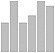vertical bar chart
bar([7 3 8 5 5])
set(gca, 'XTick', 1:5, ...
'XTickLabel', {'a', 'b', 'c', 'd', 'e'})
cnts = c(7,3,8,5,5)
names(cnts) = c("a","b","c","d","e")
barplot(cnts)

# ggplot2:
cnts = c(7,3,8,5,5)
names = c("a","b","c","d","e")
df = data.frame(names, cnts)
qplot(names, data=df, geom="bar", weight=cnts)
cnts = [7,3,8,5,5]
plt.bar(range(0,len(cnts)), cnts)
bar chart with error bars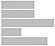horizontal bar chart
barh([7 3 8 5 5])cnts = c(7,3,8,5,5)
names(cnts) = c("a","b","c","d","e")
barplot(cnts, horiz=T)
cnts = [7,3,8,5,5]
plt.barh(range(0,len(cnts)), cnts)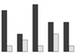grouped bar chart
d = [7 1; 3 2; 8 1; 5 3; 5 1]
bar(d)
data = matrix(c(7,1,3,2,8,1,5,3,5,1),
nrow=2)
labels = c("a","b","c","d","e")
barplot(data, names.arg=labels, beside=TRUE)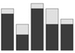stacked bar chart
d = [7 1; 3 2; 8 1; 5 3; 5 1]
bar(d, 'stacked')
data = matrix(c(7,1,3,2,8,1,5,3,5,1),
nrow=2)
labels = c("a","b","c","d","e")
barplot(data, names.arg=labels)
a1 = [7,3,8,5,5]
a2 = [1,2,1,3,1]
plt.bar(range(0,5), a1, color='r')
plt.bar(range(0,5), a2, color='b')pie chart
labels = {'a','b','c','d','e'}
pie([7 3 8 5 5], labels)
cnts = c(7,3,8,5,5)
names(cnts) = c("a","b","c","d","e")
pie(cnts)
cnts = [7,3,8,5,5]
labs = ['a','b','c','d','e']
plt.pie(cnts, labels=labs)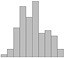histogram
hist(randn(1, 100), 10)hist(rnorm(100), breaks=10)

hist(rnorm(100), breaks=seq(-3, 3, 0.5))

# ggplot2:
x = rnorm(50)
binwidth = (max(x) - min(x)) / 10
qplot(x, geom="histogram", binwidth=binwidth)
plt.hist(sp.randn(100),
bins=range(-5,5))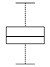box plot
boxplot(randn(1, 100))boxplot(rnorm(100))plt.boxplot(sp.randn(100))
box plots side-by-sideboxplot([randn(1, 100)
exprnd(1, 1, 100)
unifrnd(0, 1, 1, 100)]')
boxplot(rnorm(100), rexp(100), runif(100))plt.boxplot([sp.randn(100),
np.random.uniform(size=100),
np.random.exponential(size=100)])
scatter plots
matlabrnumpyjulia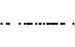strip chart
data = randn(1, 50)
plot(data, zeros(size(data)), 'o')
stripchart(rnorm(50))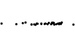strip chart with jitter
stripchart(floor(50 * runif(20)),
method="jitter")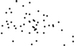scatter plot
plot(randn(1,50),randn(1,50),'+')plot(rnorm(50), rnorm(50))plt.scatter(sp.randn(50), sp.randn(50), marker='x')plot(randn(20), randn(20), '.k', randn(20), randn(20), '.r')plot(rnorm(20), rnorm(20))
points(rnorm(20) + 1, rnorm(20) + 1,
col='red')
point types'.': point
'o': circle
'x': x-mark
'+': plus
'*': star
's': square
'd': diamond
'v': triangle (down)
'^': triangle (up)
'<': triangle (left)
'>': traingle (right)
'p': pentagram
'h': hexagram
Integer values for pch parameter:

0: open square
1: open circle
2: open triangle, points up
3: cross
4: x
5: open diamond
6: open triangle, points down
15: solid square
16: solid circle
17: solid triangle, points up
18: solid diamond
marker parameter takes these string values:

'.': point
',': pixel
'o': circle
'v': triangle_down
'^': triangle_up
'<': triangle_left
'>': triangle_right
'1': tri_down
'2': tri_up
'3': tri_left
'4': tri_right
'8': octagon
's': square
'p': pentagon
'*': star
'h': hexagon1
'H': hexagon2
'+': plus
'x': x
'D': diamond
'd': thin_diamond
'|': vline
'_': hline
point sizeplot(rnorm(50), rnorm(50), cex=2)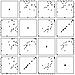scatter plot matrix
x = rnorm(20)
y = rnorm(20)
z = x + 3*y
w = y + 0.1*rnorm(20)
df = data.frame(x, y, z, w)

pairs(df)3d scatter plot
install.packages('scatterplot3d')
library('scatterplot3d')

scatterplot3d(rnorm(50), rnorm(50),
rnorm(50), type="h")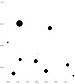bubble chart
install.packages('ggplot2')
library('ggplot2')

df = data.frame(x=rnorm(20),
y=rnorm(20), z=rnorm(20))

p = ggplot(df, aes(x=x, y=y, size=z))
p + geom_point()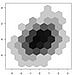hexagonal bins
install.packages('hexbin')
library('hexbin')

plot(hexbin(rnorm(1000),
rnorm(1000),
xbins=12))
hexbin(randn(1000),
randn(1000),
gridsize=12)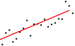linear regression line
x = 0:20
y = 2 * x + rnorm(21) * 10

fit = lm(y ~ x)

plot(y)

# Distributions

A distribution density function f(x) is a non-negative function which, when integrated over its entire domain is equal to one. The distributions described in this sheet have as their domain the real numbers. The support of a distribution is the part of the domain on which the density function is non-zero.

A distribution density function can be used to describe the values one is likely to see when drawing an example from a population. Values in areas where the density function is large are more likely than values in areas where the density function is small. Values where there density function is zero do not occur. Thus it can be useful to plot the density function.

To derive probabilities from a density function one must integrate or use the associated cumulative density function

\begin{align} F(x) = \int_{-\infty}^x f(t) dt \end{align}

which gives the probability of seeing a value less than or equal to x. As probabilities are non-negative and no greater than one, F is a function from (-, ) to [0,1]. The inverse of F is called the inverse cumulative distribution function or the quantile function for the distribution.

For each distribution statistical software will generally provide four functions: the density, the cumulative distribution, the quantile, and a function which returns random numbers in frequencies that match the distribution. If the software does not provide a random number generating function for the distribution, the quantile function can be composed with the built-in random number generator that most languages have as long as it returns uniformly distributed floats from the interval [0, 1].

 density probability density probability mass cumulative density cumulative distribution distribution inverse cumulative density inverse cumulative distribution quantile percentile percent point random variate

Discrete distributions such as the binomial and the poisson do not have density functions in the normal sense. Instead they have probability mass functions which assign probabilities which sum up to one to the integers. In R warnings will be given if non integer values are provided to the mass functions dbinom and dpoiss.

The cumulative distribution function of a discrete distribution can still be defined on the reals. Such a function is constant except at the integers where it may have jump discontinuities.

Most well known distributions are in fact parametrized families of distributions. This table lists some of them with their parameters and properties.

The information entropy of a continuous distribution with density f(x) is defined as:

\begin{align} -\int_\mathbb{R} f(x) \; \log(f(x)) \; dx \end{align}

In Bayesian analysis the distribution with the greatest entropy, subject to the known facts about the distribution, is called the maximum entropy probability distribution. It is considered the best distribution for modeling the current state of knowledge.

## binomial

The probability mass, cumulative distribution, quantile, and random number generating functions for the binomial distribution.

The binomial distribution is a discrete distribution. It models the number of successful trails when n is the number of trials and p is the chance of success for each trial. An example is the number of heads when flipping a coin 100 times. If the coin is fair then p is 0.50.

numpy:

Random numbers in a binomial distribution can also be generated with:

np.random.binomial(n, p)


## poisson

The probability mass, cumulative distribution, quantile, and random number generating functions for the Poisson distribution.

The Poisson distribution is a discrete distribution. It is described by a parameter lam which is the mean value for the distribution. The Poisson distribution is used to model events which happen at a specified average rate and independently of each other. Under these circumstances the time between successive events will be described by an exponential distribution and the events are said to be described by a poisson process.

numpy:

Random numbers in a Poisson distribution can also be generated with:

np.random.poisson(lam, size=1)


## normal

The probability density, cumulative distribution, quantile, and random number generating functions for the normal distribution.

The parameters are the mean μ and the standard deviation σ. The standard normal distribution has μ of 0 and σ of 1.

The normal distribution is the maximum entropy distribution for a given mean and variance. According to the central limit theorem, if {X1, …, Xn} are any independent and identically distributed random variables with mean μ and variance σ2, then Sn := Σ Xi / n converges to a normal distribution with mean μ and variance σ2/n.

numpy:

Random numbers in a normal distribution can also be generated with:

np.random.randn()


## gamma

The probability density, cumulative distribution, quantile, and random number generating functions for the gamma distribution.

The parameter k is called the shape parameter and θ is called the scale parameter. The rate of the distribution is β = 1/θ.

If Xi are n independent random variables with Γ(ki, θ) distribution, then Σ Xi has distribution Γ(Σ ki, θ).

If X has Γ(k, θ) distribution, then αX has Γ(k, αθ) distribution.

## exponential

The probability density, cumulative distribution, quantile, and random number generating functions for the exponential distribution.

## chi-squared

The probability density, cumulative distribution, quantile, and random number generating functions for the chi-squared distribution.

## beta

The probability density, cumulative distribution, quantile, and random number generating functions for the beta distribution.

## uniform

The probability density, cumulative distribution, quantile, and random number generating functions for the uniform distribution.

The uniform distribution is described by the parameters a and b which delimit the interval on which the density function is nonzero.

The uniform distribution the is maximum entropy probability distribution with support [a, b].

Consider the uniform distribution on [0, b]. Suppose that we take k samples from it, and m is the largest of the samples. The minimum variance unbiased estimator for b is

\begin{align} \frac{k+1}{k}m \end{align}

octave, r, numpy:

a and b are optional parameters and default to 0 and 1 respectively.

## Student's t

The probability density, cumulative distribution, quantile, and random number generating functions for Student's t distribution.

## Snedecor's F

The probability density, cumulative distribution, quantile, and random number generating functions for Snedecor's F distribution.

## empirical density function

How to construct a density function from a sample.

# Linear Regression

## simple linear regression

How to get the slope a and intercept b for a line which best approximates the data. How to get the residuals.

If there are more than two data points, then the system is overdetermined and in general there is no solution for the slope and the intercept. Linear regression looks for line that fits the points as best as possible. The least squares solution is the line that minimizes the sum of the square of the distances of the points from the line.

The residuals are the difference between the actual values of y and the calculated values using ax + b. The norm of the residuals can be used as a measure of the goodness of fit.

# Statistical Tests

A selection of statistical tests. For each test the null hypothesis of the test is stated in the left column.

In a null hypothesis test one considers the p-value, which is the chance of getting data which is as or more extreme than the observed data if the null hypothesis is true. The null hypothesis is usually a supposition that the data is drawn from a distribution with certain parameters.

The extremeness of the data is determined by comparing the expected value of a parameter according to the null hypothesis to the estimated value from the data. Usually the parameter is a mean or variance. In a one-tailed test the p-value is the chance the difference is greater than the observed amount; in a two-tailed test the p-value is the chance the absolute value of the difference is greater than the observed amount.

Octave and MATLAB have different names for the statistical test functions. The sheet shows the Octave functions; the corresponding MATLAB functions are:

octavematlab
wilcoxon_testranksum
kruskal_wallis_testkruskalwallis
kolmogorov_smirnov_testkstest
kolmogorov_smirnov_test_2kstest2
t_testttest
t_test_2ttest2

## wilcoxon signed-rank test

matlab

wilcoxon_test() is an Octave function. The MATLAB function is ranksum().

## kolmogorov-smirnov test

Test whether two samples are drawn from the same distribution.

matlab:

kolmogorov_smirnov_test_2() and kolmogorov_smirnov_test() are Octave functions. The corresponding MATLAB functions are kstest2() and kstest().

kolmogorov_smirnov_test() is a one sample test; it tests whether a sample is drawn from one of the standard continuous distributions. A one sample KS test gives a repeatable p-value; generating a sample and using a two sample KS test does not.

x = randn(100, 1)

% null hypothesis is true:
kolmogorov_smirnov_test(x, "norm", 0, 1)

% alternative hypothesis is true:
kolmogorov_smirnov_test(x, "unif", -0.5, 0.5)


r:

## independent two-sample t-test

Test whether two normal variables have same mean.

r:

If the normal variables are known to have the same variance, the variance can be pooled to estimate standard error:

t.test(x, y, var.equal=T)


If the variance cannot be pooled, then Welch's t-test is used. This uses a lower (often non-integral) degrees-of-freedom value, which in turn results in a higher p-value.

## bartlett's test

A test whether variables are drawn from normal distributions with the same variance.

## levene's test

A test whether variables are drawn from distributions with the same variance.

# Time Series

A time series is a sequence of data points collected repeatedly on a uniform time interval.

A time series can be represented by a dictionary which maps timestamps to the type of the data points. A more efficient implementation exploits the fact that the time interval is uniform and stores the data points in an array. To recover the timestamps of the data points, the timestamp of the first data point and the length of the time interval are also stored.

## time series

How to create a time series from an array.

## monthly time series

How to create a time series with one data point per month.

## lookup by time

How to get to a data point in a time series by when the data point was collected.

## lookup by position in series

How to get a data point in a time series by its ordinal position.

# Bar Charts

## vertical bar chart

A chart in which numerical values are represented by horizontal bars. The bars are aligned at the bottom.

## horizontal bar chart

A bar chart with horizontal bars which are aligned on the left.

## grouped bar chart

Optionally data sets with a common set of labels can be charted with a grouped bar chart which clusters the bars for each label. The grouped bar chart makes it easier to perform comparisons between labels for each data set.

## stacked bar chart

Two or more data sets with a common set of labels can be charted with a stacked bar chart. This makes the sum of the data sets for each label readily apparent.

## pie chart

A pie chart displays values using the areas of circular sectors or equivalently the lengths of the arcs of those sectors.

A pie chart implies that the values are percentages of a whole.

## histogram

A histogram is a bar chart where each bar represents a range of values that the data points can fall in. The data is tabulated to find out how often data points fall in each of the bins and in the final chart the length of the bars corresponds to the frequency.

A common method for choosing the number of bins using the number of data points is Sturges' formula:

\begin{align} \lceil \log_2{x} + 1 \rceil \end{align}

## box plot

Also called a box-and-whisker plot.

The box shows the locations of the 1st quartile, median, and 3rd quartile. These are the same as the 25th percentile, 50th percentile, and 75th percentile.

The whiskers are sometimes used to show the maximum and minimum values of the data set. Outliers are sometimes shown explicitly with dots, in which case all remaining data points occur inside the whiskers.

r:

How to create a box plot with ggplot2:

qplot(x="rnorm", y=rnorm(50), geom="boxplot")

qplot(x=c("rnorm", "rexp", "runif"), y=c(rnorm(50), rexp(50), runif(50)), geom="boxplot")


# Scatter Plots

## scatter plot

A scatter plot can be used to determine if two variables are correlated.

r:

How to make a scatter plot with ggplot:

x = rnorm(50)
y = rnorm(50)
p = ggplot(data.frame(x, y), aes(x, y))
p = p + layer(geom="point")
p


## hexagonal bins

A hexagonal binning is the two-dimensional analog of a histogram. The number of data points in each hexagon is tabulated, and then color or grayscale is used to show the frequency.

A hexagonal binning is superior to a scatter-plot when the number of data points is high because most scatter-plot software doesn't indicate when points are occur on top of each other.

## linear regression line

How to plot a line determined by linear regression on top of a scatter plot.

## quantile-quantile plot

Also called a Q-Q plot.

A quantile-quantile plot is a scatter plot created from two data sets. Each point depicts the quantile of the first data set with its x position and the corresponding quantile of the second data set with its y position.

If the data sets are drawn from the same distribution then most of the points should be close to the line y = x. If the data sets are drawn from distributions which have a linear relation then the Q-Q plot should also be close to linear.

If the two data sets have the same number of elements, one can simply sort them and create the scatterplot.

If the number of elements is different, one generates a set of quantiles (such as percentiles) for each set. The quantile function of MATLAB and R is convenient for this. With Python, one can use scipy.stats.scoreatpercentile.

# Line Charts

## polygonal line plot

How to connect the dots of a data set with a polygonal line.

How to add another line to a plot.

## function plot

How to plot a function.

# Chart Options

## chart title

How to set the chart title.

r:

The qplot commands supports the main options for setting the title:

qplot(x="rnorm", y=rnorm(50), geom="boxplot", main="boxplot example")


## axis labels

How to label the x and y axes.

r:

How to label the axes with ggplot2:

x = rnorm(20)
y = x^2

p = ggplot(data.frame(x, y), aes(x, y))
p + layer(geom="point") + xlab('x') + ylab('x squared')


## axis limits

How to manually set the range of values displayed by an axis.

## colors

How to set the color of points and lines.

## superimposed plots with different y-axis scales

How to superimpose two plots with different y-axis scales.

To minimize the risk that the reader will read off an incorrect y-value for a data point, the example uses the same color for the y-axis as it does for the corresponding data set.

## legend

How to put a legend on a chart.

r:

These strings can be used as the first argument to control the legend position:

• "bottomright"
• "bottom"
• "bottomleft"
• "left"
• "topleft"
• "top"
• "topright"
• "right"
• "center"

The named parameter lwd is the line width. It is roughly the width in pixels, though the exact interpretation is device specific.

The named parameter lty specifies the line type. The value can be either an integer or a string:

numberstring
0'blank'
1'solid'
2'dashed'
3'dotted'
4'dotdash'
5'longdash'
6'twodash'

# MATLAB

The basic data type of MATLAB is a matrix of floats. There is no distinction between a scalar and a 1x1 matrix, and functions that work on scalars typically work on matrices as well by performing the scalar function on each entry in the matrix and returning the results in a matrix with the same dimensions. Operators such as the logical operators ('&' '|' '!'), relational operators ('==', '!=', '<', '>'), and arithmetic operators ('+', '-') all work this way. However the multiplication '*' and division '/' operators perform matrix multiplication and matrix division, respectively. The .* and ./ operators are available if entry-wise multiplication or division is desired.

Floats are by default double precision; single precision can be specified with the single constructor. MATLAB has convenient matrix literal notation: commas or spaces can be used to separate row entries, and semicolons or newlines can be used to separate rows.

Arrays and vectors are implemented as single-row (1xn) matrices. As a result an n-element vector must be transposed before it can be multiplied on the right of a mxn matrix.

Numeric literals that lack a decimal point such as 17 and -34 create floats, in contrast to most other programming languages. To create an integer, an integer constructor which specifies the size such as int8 and uint16 must be used. Matrices of integers are supported, but the entries in a given matrix must all have the same numeric type.

Strings are implemented as single-row (1xn) matrices of characters. Matrices cannot contain strings. If a string is put in matrix literal, each character in the string becomes an entry in the resulting matrix. This is consistent with how matrices are treated if they are nested inside another matrix. The following literals all yield the same string or 1xn matrix of characters:

'foo'
[ 'f' 'o' 'o' ]
[ 'foo' ]
[ [ 'f' 'o' 'o' ] ]


true and false are functions which return matrices of ones and zeros. The ones and zeros have type logical instead of double, which is created by the literals 1 and 0. Other than having a different class, the 0 and 1 of type logical behave the same as the 0 and 1 of type double.

MATLAB has a tuple type (in MATLAB terminology, a cell array) which can be used to hold multiple strings. It can also hold values with different types.

# R

The primitive data types of R are vectors of floats, vectors of strings, and vectors of booleans. There is no distinction between a scalar and a vector with one entry in it. Functions and operators which accept a scalar argument will typically accept a vector argument, returning a vector of the same size with the scalar operation performed on each the entries of the original vector.

The scalars in a vector must all be of the same type, but R also provides a list data type which can be used as a tuple (entries accessed by index), record (entries accessed by name), or even as a dictionary.

In addition R provides a data frame type which is a list (in R terminology) of vectors all of the same length. Data frames are equivalent to the data sets of other statistical analysis packages.

# NumPy

NumPy is a Python library which provides a data type called array. It differs from the Python list data type in the following ways:

• N-dimensional. Although the list type can be nested to hold higher dimension data, the array can hold higher dimension data in a space efficient manner without using indirection.
• homogeneous. The elements of an array are restricted to be of a specified type. The NumPy library introduces new primitive types not available in vanilla Python. However, the element type of an array can be object which permits storing anything in the array.

In the reference sheet the array section covers the vanilla Python list and the multidimensional array section covers the NumPy array.

List the NumPy primitive types

SciPy, Matplotlib, and Pandas are libraries which depend on Numpy.

# Julia

http://julialang.org/